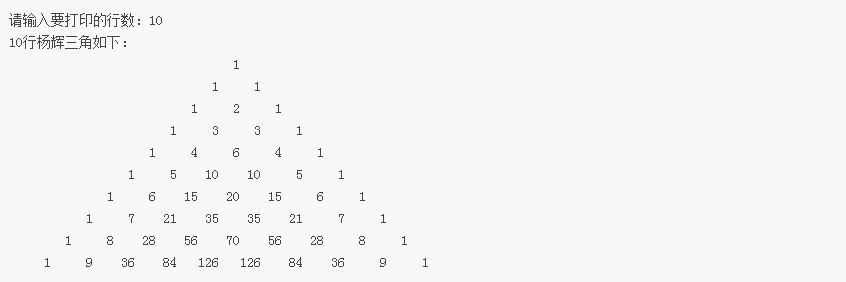2021-07-21 15:55

# 杨辉三角问题C语言，今天

• 写回答
• 关注问题
• 收藏
• 邀请回答

#### 2条回答默认 最新

•已采纳
``````#include <stdio.h>
#include <stdlib.h>
#define N 10
void main()
{
while(1)
{
system("cls");
int i, j, k, n=0, a[N][N];  /*定义二维数组a*/
printf("请输入要打印的行数(输入<=0时结束）：");
scanf("%d",&n);
if(n<=0)
return;
if(n>10)
{
printf("行数不能大于10\n"):
continue;
}
printf("%d行杨辉三角如下：\n",n);
for(i=1;i<=n;i++)
a[i] = a[i][i] = 1;  /*两边的数令它为1，因为现在循环从1开始，就认为a[i]为第一个数*/
for(i=3;i<=n;i++)
for(j=2;j<=i-1;j++)
a[i][j]=a[i-1][j-1]+a[i-1][j];  /*除两边的数外都等于上两顶数之和*/
for(i=1;i<=n;i++){
for(k=1;k<=n-i;k++)
printf("   ");  /*这一行主要是在输出数之前打上空格占位，让输出的数更美观*/
for(j=1;j<=i;j++)  /*j<=i的原因是不输出其它的数，只输出我们想要的数*/
printf("%5d",a[i][j]);

printf("\n");  /*当一行输出完以后换行继续下一行的输出*/
}
system("pause");
}
printf("\n");
}

``````
已采纳该答案
打赏 评论
•CSDN专家-Fay 2021-07-21 15:57

这类代码有很多啊，参考一下：

``````#include <stdio.h>
#define N 14
void main()
{
int i, j, k, n=0, a[N][N];  /*定义二维数组a*/
while(n<=0||n>=13){  /*控制打印的行数不要太大，过大会造成显示不规范*/
printf("请输入要打印的行数：");
scanf("%d",&n);
}
printf("%d行杨辉三角如下：\n",n);
for(i=1;i<=n;i++)
a[i] = a[i][i] = 1;  /*两边的数令它为1，因为现在循环从1开始，就认为a[i]为第一个数*/
for(i=3;i<=n;i++)
for(j=2;j<=i-1;j++)
a[i][j]=a[i-1][j-1]+a[i-1][j];  /*除两边的数外都等于上两顶数之和*/
for(i=1;i<=n;i++){
for(k=1;k<=n-i;k++)
printf("   ");  /*这一行主要是在输出数之前打上空格占位，让输出的数更美观*/
for(j=1;j<=i;j++)  /*j<=i的原因是不输出其它的数，只输出我们想要的数*/
printf("%6d",a[i][j]);

printf("\n");  /*当一行输出完以后换行继续下一行的输出*/
}
printf("\n");
}

``````打赏 评论